## neděle 11. března 2012

### Collective Intelligence: Ants colony solving TSP

According to wikipedia:

Collective intelligence is a shared or group intelligence that emerges from the collaboration and competition of many individuals and appears in consensus decision making in bacteria, animals, humans and computer networks”.

This article describes, how to make the ants, find the solution for TSP problem. Implemented in Python. Get the source from GitHUb.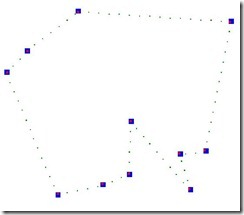The algorithms based on the collective intelligence have some “interesting” properties:

• decentralization
• parallelism
• "robustness" (failures)
• auto-organization

These algorithms are inspired by the nature. Here are some examples of collective intelligence which can be observed in the nature:

1. The swallows settle on wires before they are taking of for the next destination. There is no leader in the group. The decision whether to take of is taken collectively. The probability for the swallow to take of is getting higher when there are more swallows in the air. If the other swallows do not join, the swallow will again settle down on the wire. At one point the number of the swallows in the air reaches the “break-point” when all the swallows decide to take of.
2. The bees perform a special “dance” to show their peers where the foot-source is. This dance gives the information about the angle of the food source position with respect to the sun. All the bees do perform the dance when coming back in, which makes the algorithm adaptive.
3. When the ant founds food, he lays down a "positive pheromone" on his way back. This pheromone evaporates during the time. The other ants sniff for this pheromone when choosing their route and prefer to go in places where the concentration of the pheromone is higher. The shorter the path to the food source is, more pheromone stays on the track before it evaporates. The more pheromone there is, more ants take this path. When there is a obstacle in the route – the algorithm adapts easily to knew situation. Again the shortest route to evict the obstacle is chosen in the shortest time. Some details here.

There exist several applications of collective intelligence in engineering and optimization. The ants example has applications specially in routing. One of the basic problems which can be solved using an Ant colony is Travelling Salesman Problem.

### Travelling Salesman Problem

Travelling Salesman Problem is a classical problem in the field of graph theory. Given n cities the salesman has to visit all of the nodes, come back to his starting location and and minimize traveled distance. Although the problem is NP - hard, several heuristic algorithms exists which obtain suitable results in polynomial time.

### Ant colony algorithm for TSP

Ant colony algorithm was introduced in year 1997 by Italian researcher Marco Dorigo.

On the beginning the ants start to explore the graph. They choose their nodes randomly, until they visit all of the nodes. A this point the ant starts to copy his path back to his starting point. While he copies the path, on each edge he lays down certain amount of pheromone inversely proportional to the length of the tour. When each ant starts new route in each node he will compute the probability to choose an edge to continue his route. The probability of choosing edge e in each step is computed as follows.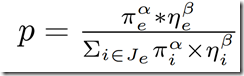• π_e  corresponds to the value of pheromone on the edge e.
• η_e corresponds to the quality of the route. We can estimate this value by the length of the edge η_e = 1/d_e where d_e is the length of the edge.
• J_e is a set of all the edges which the ant can use for his next step - includes all the edges except the one for which we compute the probability.
• α and β are coefficients used to manage the impact of the length of the finished route to affect the decision other ants.
• The amount of pheromone given to a certain edge is l = 1/routeLength^k, where k is a coefficient to amplify the impact of the length of the route to the decision.
• During the time the pheromone evaporates on the edges. The evaporation can be expressed as: π_e = (1-ρ)π_e

### The implementation in Python

Most of what I have learned and presented here was done during Collective Intelligence intensive course at Telecom ParisTech done by Jean-Luis Dessales, being part of the Athens program. The implementation was done in Python but as a module to a EvoLife program, which is a custom tool developed by Jean-Luis Dessales for scientific observations on genetic algorithms and collective intelligence algorithms. I have decided to make a stand-alone python program by taking the important bits out just for the Ants colony algorithm. I do almost never work in python, so for anyone out there if you see any big wrongdoing against the python culture, naming conventions etc, let me know.

The most important bits are in the Ant class.

```self.Action = 'SearchNextNode'
self.Node
self.Path = []
self.PathLength = 0
self.ToVisit = []
self.Visited = []
```

The Action field remembers the Ant’s actual state. Each action has a corresponding method associated with it. The Node field holds the actual field. In ToVisit and Visited the ant stores the Nodes that he had already visited or that he needs to visit in order to achieve TSP completeness. Here is the “move” method which is called repetitively for each ant:

```def moves(self):
#here is the ants move - one of the following actions is always selected
if self.Action == 'GoToNode':
self.goToNode()
if self.Action == 'SearchNextNode':
self.searchNextNode()
if self.Action == 'ReturnToStart':
self.returnToStart()
if self.Action == "GoToFirst":
self.goToFirst()```

The most important of these method is searchNextNode, where the ant searches for his next edges to explore. In this method the behavior described in previous paragraph has to be defined.

```def searchNextNode(self):
nodeindex = self.Node.Id
#the maximal probability
pMax = 0
p = 0

#Try to select the best node by the pheromones in the direction
#have to iterate over all nodes
for i in range(NbNodes):
if i!=self.Node.Id and self.Visited[i]==0:
d = Land.Distances[self.Node.Id][i]

#get the value of pheromon on the edge
pi = Land.Edges[self.Node.Id][i]

#To prevent division by zero and get some info
#when d = 0 there would be problem in computation of d
if d==0:
print i
print self.Node.Id

#the quality of the route
nij = 1/d

pselected = math.pow(pi,alfa) * math.pow(nij,beta)

#normalization
#compute the sum of other options
sum = 0
for j in range(NbNodes):
if j!=self.Node.Id and self.Visited[j]==0 and j!=i:
dj = Land.Distances[self.Node.Id][j]
pij = Land.Edges[self.Node.Id][j]
nj = 1/dj
pj = math.pow(pij,alfa) * math.pow(nj,beta)
sum+=pj
if sum>0:
p = pselected/sum

#if we have a new best path - then remember the index
if p > pMax:
pMax = p
nodeindex = i
```

We have to iterate over all the neighbor nodes in order to choose the right one. For each node the probability of taking the edge going to this node is computed according to the formula given in previous paragraph. Some remarks: the value of the pheromone on each edge is stored in a global array:  Land.Edge[nodeFrom][nodeTo]. Also the distances between all nodes are pre-calculated in Land.Distance[nodeFrom][nodeTo].

There is quite a lot of code, regarding the visualisation. The TkInter library was used for drawing. Also the PIL library has to be installed. It should not take too long the figure out the responsibility of each class.

### The results

Here is how the resulting program looks like: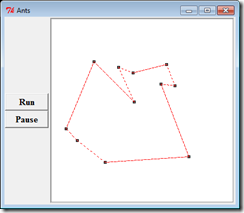And here is the evolution of creating the final decision. First all edges have some amount of pheromone and during the time, the preferred edges are chosen. Because the ants choose the edges randomly on the beginning, the result is never assumed the same. The following three images show the evolution which resulted in quite not optimal solution.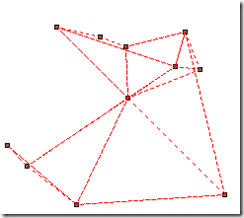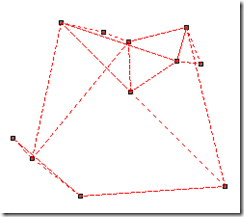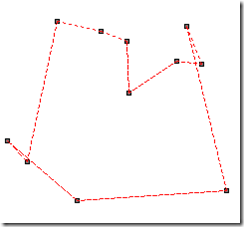The quality of the solution depends heavily on the values of the coefficients. These values can be changed in the Program.py file:

```#level of pheromone to show
PToShow = 0.004
#factor which lowers the value given to a path on function of the paths length
k=1
#evaporation factor
theta = 0.07
#parameter which amplifies the value of the pheromon on the edge (pi^alfa)
alfa = 4
#parameter which amplifies the impact of the quality of the route  ni^beta; ni=1/de
beta = 2.5```
Putting aside the coefficients described above, there is also PToShow value, which determines what is the minimal value of pheromone on the edge, in order for the edge to be dotted in the picture.

Before this implementation, I had one before – which was not at all efficient but quite funny. In this implementation the ants, could move freely around – there was no notion of edges. The ants simply took a directions towards a certain node and when they got close enough to it, they considered the node as reached and continued for the other one. This was useless, but I saved these funny graphics with the ants moving all around: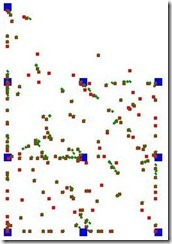And the ants finding the solution: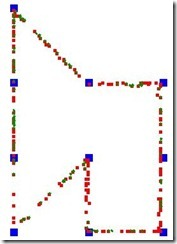### The source code

1.